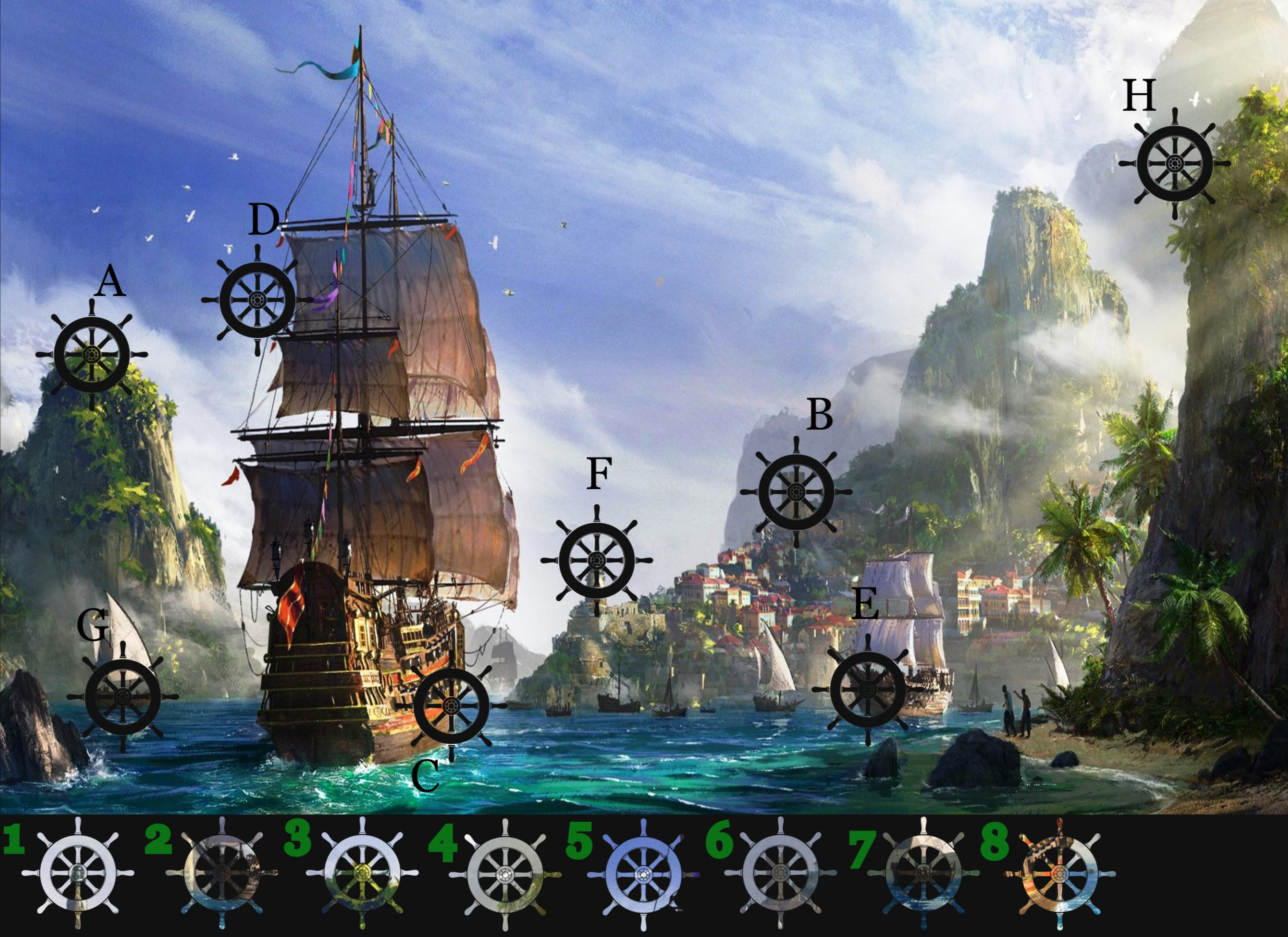# ClosedForge Ahoy!

Status
Not open for further replies.

#### DeletedUser4727

The brutal pirate Bloodbeard has been causing havoc again! Within the city, he has defaced the Pirate's image in the local tavern, Bloodbeard has stamped out the the ships helm on the picture. General Grivus has assigned pirate Jane with the task of finding out which pieces goes in to each spot, pirate Jane is pleading for your help with this challenging task.

The Black spaces have been marked with letters A to H and the loose pieces have been noted with the numbers 1 to 8.

All you need to do is match them up together for example, 1 = A, etc., Please note - this is an example and not the actual answer.Only one entry per account

How to take part and enter the competition.

1. Scroll down to the bottom you will see a text box
3. Click enter

The competition will close at 23:59 on Monday 10th September 2018. Your answers will be hidden so that other players can not see your answers and copy. Therefore your post will say "awaiting moderators approval". Once the competition has closed your answers will be reviewed and all posts will be verified and the winners will be posted. There will be 2 winners chosen at random who will receive 1,000 Diamonds each!

1-F
2-E
3-A
4-H
5-D
6-B
7-G
8-C

A = 3
B = 6
C = 8
D = 5
E = 2
F = 1
G = 7
H = 4

Hi

A3
B6
C8
D5
E2
F1
G7
H4

Cheers

1 = F
2 = E
3 = A
4 = H
5 = D
6 = B
7 = G
8 = C

1=F
2=E
3=A
4=H
5=D
6=B
7=G
8=C

1 = F
2 = E
3 = A
4 = H
5 = D
6 = B
7 = G
8 = C

1=F
2=E
3=A
4=H
5=D
6=B
7=G
8=C

1 + F

2 = E

3 = A

4 = H

5 = D

6 = B

7 = G

8 = C

#### Deep the Fried Potato

##### Tsar
Heyoo mateys. Here are my answers..

1-F
2-E
3-A
4-H
5-D
6-B
7-G
8-C

#### DeletedUser112513

A3, B6, C8, D5, E2, F1, G7, H4

#### DeletedUser114704

1 = F, 2 = E, 3=A, 4=H, 5=D, 6=B, 7=G, 8=C.

A3
B6
C8
D5
E2
F1
G7
H4

1 = F
2 = E
3 = A
4 = H
5 = D
6 = B
7 = G
8 = C

A-3
B-6
C-8
D-5
E-2
F-1
G-7

#### DeletedUser114606

1=F, 2=E, 3=A, 4=H, 5=D, 6=B, 7=G, 8=C

#### DeletedUser113215

a3, b6, c8, d5, e2, f1, g7, h4

1 = F
2 = G
3 = A
4 = H
5 = D
6 = B
7 = G
8 = C

A = 3
B = 6
C = 8
D = 5
E = 2
F = 1
G = 7
H = 4

#### DeletedUser112754

1-F, 2-E, 3-A, 4-H, 5-D, 6-B, 7-G, 8-C

Status
Not open for further replies.